## Petrophysics: Gamma Ray Normalization in Python

Normalization of well log data is a common and routine process within a petrophysical workflow and is used to correct for variations in logging curves between wells. These variations can arise due a number of different reasons such as incorrect tool calibrations, varying tool vintage and changes in borehole environmental conditions between the wells.

• What normalization is

• Why we want to normalize well log data

• How we carry out normalization

• Example of normalization within Python

### What is normalization?

Normalization is the process of re-scaling or re-calibrating the well logs so that they are consistent with other logs in other wells within the field or region. This can be achieved by applying a single point normalization (linear shift) or a two point normalization (‘stretch and squeeze’) to the required curve.

Normalization is commonly applied to gamma ray logs, but can be applied to neutron porosity, bulk density, sonic and spontaneous potential logs as well. Resistivity logs are generally not normalized unless there is a sufficient reason to do so (Shier, 2004).

You should discuss with your stratigrapher/geologist prior to carrying out normalization and also carry out a thorough QC of your data to understand if it is necessary. Applying normalization blindly to your data can result in geological variation and features being removed from the data. Therefore, it should be considered carefully. Shier (2004) provides an excellent discussion and guidelines on how to carry out normalization on well log data and is well worth reviewing.

### Why do we want to normalize our well log data?

Within a region, it is commonly assumed that similar geological and stratigraphical units should show similar minimum and maximum log values and also similar log responses. However, there are a multitude of reasons why the log responses may differ from the expected pattern between the logged wells. These can include:

• Differences in borehole environment such as lithological variations

• Differences in wellbore shape affecting the measurements, for example an enlarged section of the borehole will have more borehole fluid in it and this can lead to an attenuation of gamma ray logs, which in turn leads to a reduction in values

• Incorrectly applied tool calibrations

• Variations in logging tool technology and sensors through time

• Diagenetic changes to the rock

• Drift in the tool responses

• Different tools from multiple service companies

Carrying out normalization allows us to make useful comparisons between multiple wells. It also makes batch processing more efficient, especially when selecting key interpretation parameters.

Additionally, normalization can be applied to data even if that data has been previously borehole corrected (Shier, 2004). This is useful when working with datasets where there is no knowledge of what corrections have been applied, especially in older datasets.

### How do we normalize well log data?

The workflow for normalization usually involves selecting a key well within the region or field and selecting key parameters from it and re-scaling other wells to match.

Normalization can be carried out using a simple linear shift of the log data using the equation below. This is useful where we know we have a fixed shift in the data such as when neutron porosity has been recorded in different lithology units (Crain’s Petrophysics, 2020).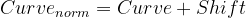More commonly a two-point shift is applied, whereby the data is stretched and squeezed to match the reference well. This is is frequently applied to gamma ray data. This is calculated as follows: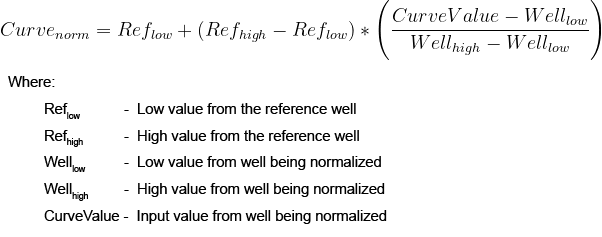The key parameters — which are selected from the reference / key well — can be the minimum or maximum values. More commonly they are values obtained from percentiles such as the 5th and 95th percentile. If using the minimum and maximum values you need to be wary of outliers and anomalous readings. For example, if the gamma ray in the reference well read a single value of 600 API and it was used as a reference maximum value it would stretch out the other wells being normalized to this range.

## Normalization Example Using Python

The following code walkthrough shows one way of normalizing well log data using a pandas dataframe and three wells from the Volve Dataset (Equinor, 2018).

You can find the full Jupyter Notebook for this example within **8 — Curve Normalisation.ipynb **of my Petrophysics and Python series on GitHub at the following link: andymcdgeo/Petrophysics-Python-Series *This series of Jupyter Notebooks take you through various aspects of working with Python and Petrophysical data.*github.com

The first step is to import the required libraries that we plan to use. In this case it will be the pandas and matplotlib libraries.

``````    import pandas as pd
import matplotlib.pyplot as plt
``````

We can load in our data straight from CSV. In this example I am using a subset of 3 wells from the Volve dataset.

``````    data = pd.read_csv('Data/VolveWells.csv')
``````

We can then obtain details about the data with a few simple commands:

``````    data.head()
``````

This returns the column headers and the first 5 rows of the data: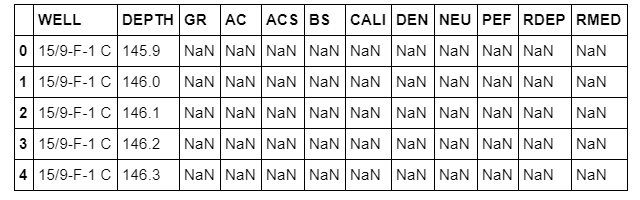We can see right away what curves we have in this dataset and that the nulls are represented by NaN (Not a Number) values. Which is good and means we don’t need to replace values.

To check what wells we have in the data we can call upon the unique() method:

``````    data['WELL'].unique()
``````

This will return an array of the well names like so:

``````    array(['15/9-F-1 C', '15/9-F-4', '15/9-F-7'], dtype=object)
``````

From this initial exploration we can say that we have:

• 3 wells within the dataset: 15/9-F-1 C, 15/9-F-4 and 15/9-F-7

• There is no need to replace any -999 values as nulls

• 10 logging curves in each well

### Plotting the Raw Data

Before we plot the data, the first step we need to do is group the dataframe by the WELL column. This will make it easier to plot on the histogram.

``````    wells = data.groupby('WELL')
``````

We can see if we call upon wells.head() we get a grouped dataframe showing the first 5 rows from each well.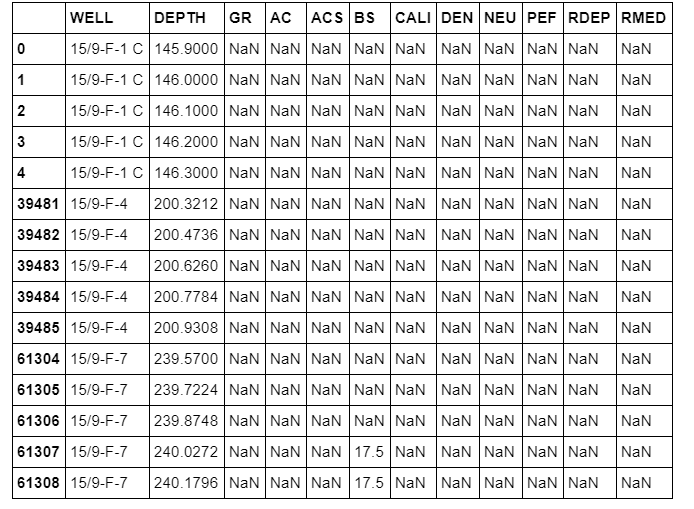One of the easiest ways to compare the distribution and select key parameters is by using a histogram. In Python, if we want to see the distribution line rather than bars we have to call upon the Kernel Density Estimation (KDE) plot as opposed to the histogram. Using our grouped dataframe allows us to loop through each well, add the data to the plot and display the correct label in the legend:

``````    fig, ax = plt.subplots(figsize=(8,6))
for label, df in wells:
df.GR.plot(kind ='kde', ax=ax, label=label)
plt.xlim(0, 200)
plt.grid(True)
plt.legend()
plt.show()
``````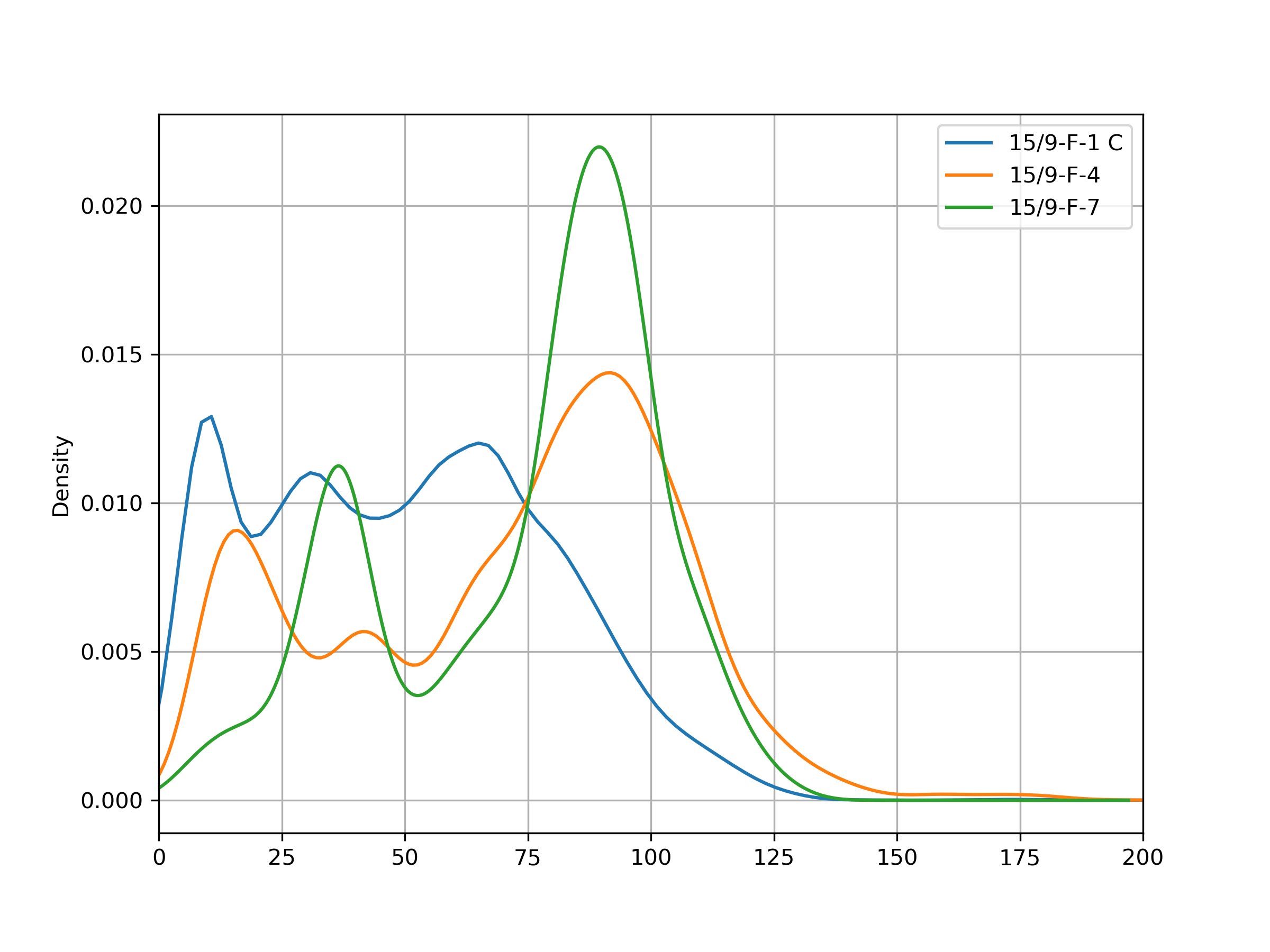From the plot above, we will assume that the key well is 15/9-F-7 and we will normalize the other two wells to this one.

Note that it does appear that the distributions run off the left hand side of the plot, but it can be confirmed using the min() method no values exist below 0 for the gamma ray curve:

``````    wells.min()
``````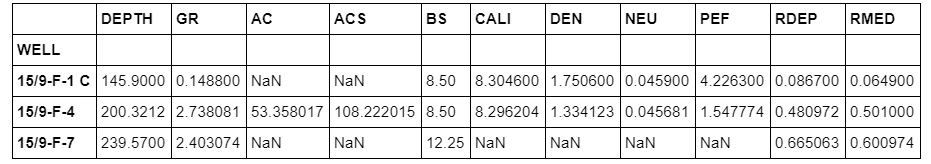### Calculating the Percentiles

As mentioned above, it is possible that datasets can contain erroneous values which may affect the minimum and the maximum values within a curve. Therefore, some interpreters prefer to base their normalization parameters on percentiles. In this example, I am going to use the 5th and 95th percentiles.

The first step is to calculate the percentile (or quantile as pandas refers to it) by grouping the data by wells and then applying the .quantile() method to a specific column. In this case, GR. The quantile function takes in a decimal value, so a value of 0.05 is equivalent to the 5th percentile and 0.95 is equivalent to the 95th percentile.

``````    gr_percentile_05 = data.groupby('WELL')['GR'].quantile(0.05)
print(gr_percentile_05)
``````

So now we need to bring that back into our main dataframe. We can do this using the .map() method, which will combine two data series that share a common column. Once it is mapped we can call upon the .describe() method and confirm that it has been added to the dataframe.

``````    data['05_PERC'] = data['WELL'].map(gr_percentile_05)
data.describe()
``````

We can see that the 5th percentile data we calculated above has now been added to the end of the dataframe. We can now repeat the above process for the 95th percentile:

``````    gr_percentile_95 = data.groupby('WELL')['GR'].quantile(0.95)
data['95_PERC'] = data['WELL'].map(gr_percentile_95)
data.describe()
``````

### Creating the Normalization Function

Before we normalize our data, we must first create a function that can be called upon multiple times. As a reminder, the function we are using is as follows (Shier, 2004):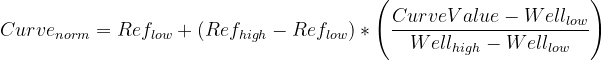``````    def normalise(curve, ref_low, ref_high, well_low, well_high):
return ref_low + ((ref_high - ref_low) * ((curve - well_low) / (well_high - well_low)))
``````

Using the percentiles calculated in the previous step, we can set our reference high and low values:

``````    key_well_low = 25.6464
key_well_high = 110.5413
``````

To apply the function to each value and use the correct percentiles for each well we can use the .apply() method to the pandas dataframe and then a lamda function for our custom function.

``````    data['GR_NORM'] = data.apply(lambda x: normalise(x['GR'], key_well_low, key_well_high, x['05_PERC'], x['95_PERC']), axis=1)
``````

### Plotting the Normalized Data

To view our final normalized data, we can re-use the code from above to generate the histogram. When we do, we can see that all curves have been normalized to our reference well.

``````    fig, ax = plt.subplots(figsize=(8,6))
for label, df in wells:
df.GR_NORM.plot(kind ='kde', ax=ax, label=label)
plt.xlim(0, 200)
plt.grid(True)
plt.legend()
plt.show()
``````

We now have our normalized gamma ray data. When compared side by side we can see the difference the normalization has made to the data.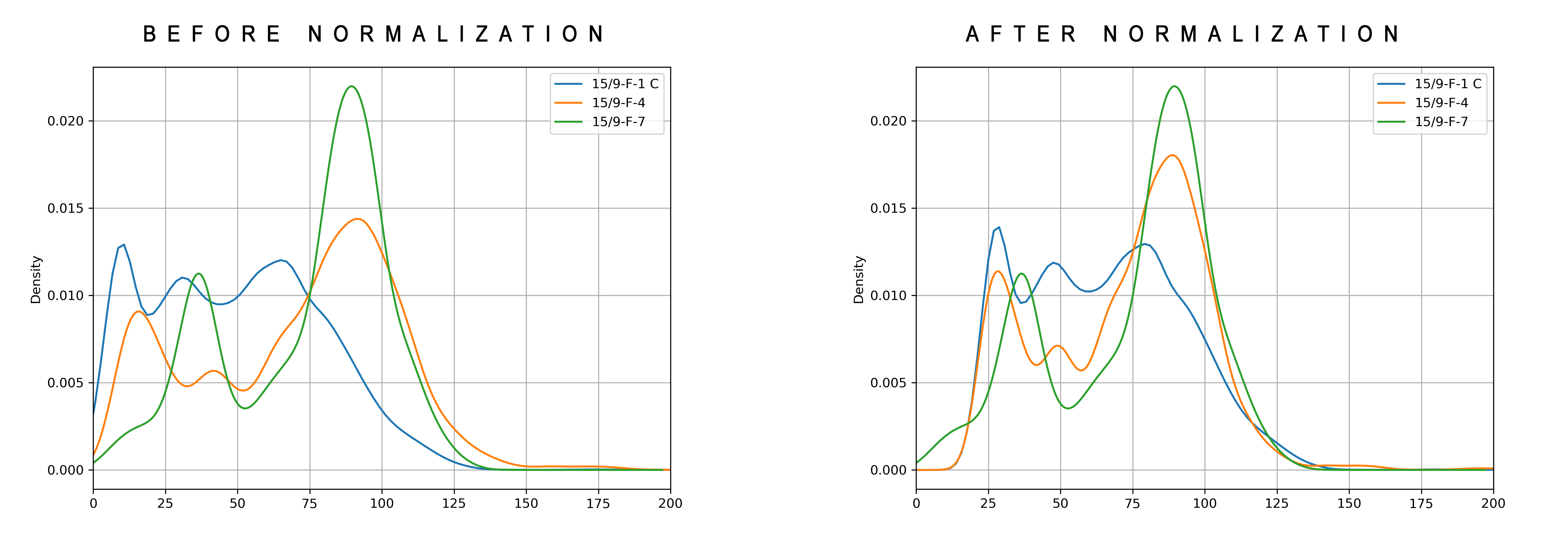## Conclusion

Normalization of well log curves is an important step within the petrophysical workflow and can be required for a variety of reasons including: poor tool calibrations, varying tool vintages, lithological differences, and borehole environmental conditions.

Carrying out normalization allows easier comparison between multiple wells and easier selection of key interpretation parameters when carrying out batch processing.

In this article we have covered how to carry out a simple normalization of a gamma ray log using Python. But the method can equally be applied to other well logs with the usual caveats that the data would need to be sense checked afterwards.

Crains Petrophysical Handbook (2020). Available at: https://www.spec2000.net/08-normalization.htm

Equinor. (2018). Disclosing all Volve data. Available at: https://www.equinor.com/en/news/14jun2018-disclosing-volve-data.html

Shier, D. E. (2004). Well log normalization: Methods and guidelines. Petrophysics, 45(3), 268–280.

Share on: